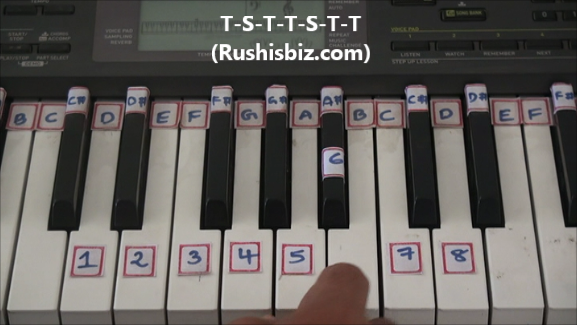HINDI - A B C D E F G H I J K L M N O P Q R S T U V W X Y Z

TAMIL - A B C D E F G H I J K L M N O P Q R S T U V W X Y Z

TELUGU - A B C D E F G H I J K L M N O P Q R S T U V W X Y Z

# ‘D’ Minor Scale Positions (Natural) – Piano Tutorials

Notes:  D-1, E-2, F-3, G-4, A-5, A#-6, C-7, D-8

Since it is a ‘D’ minor scale obviously ‘D’ will be first note, how to find the remaining notes ?

The simple formula which is used for any natural minor scale:

Tone-Semi tone-Tone-Tone-Semi tone-Tone-Tone (T-S-T-T-S-T-T)

What do you mean by Tones and Semi tones?

Tone (whole step) – If there is a one key between two notes, then the distance between those two notes is Tone or Whole step.

Eg: from D to E is a Tone or Whole step

Semi tone (half step) – If there are no keys between two notes, then the distance between those two notes is Semi tone or Half step

Eg: from A to A# is a Semi tone or Half step (Immediate keys)‘D’ Minor Scale Positions – Video Tutorials